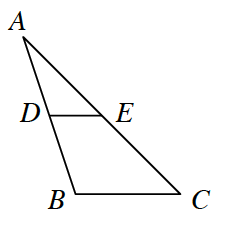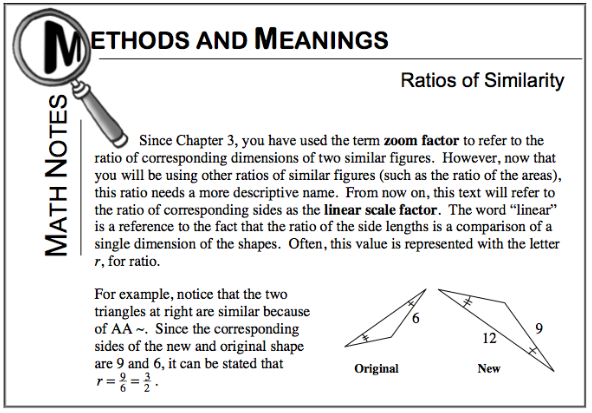Home > CCG > Chapter 9 > Lesson 9.1.3 > Problem9-33

9-33.

In the diagram below, $DE$ is a midsegment of $ΔABC$. If the area of $ΔABC$ is $96$ square units, what is the area of $ΔADE$? Explain how you know. Homework Help ✎$ΔADE\simΔABC$

Review Lesson 8.2.1, especially the Math Notes from this lesson, shown below.

What is the linear scale factor?
What is the ratio of the areas?

$24$ square units.